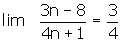Learn from home

The teachers## Exercise 1

Prove that the sequencehas a limit of 2. Also, calculate the terms whose distance from 2 is less than 0.1.

## Exercise 2

Prove that the sequencehas a limit of 4 and calculate how many terms of the succession are not within (4 − 0.001, 4 + 0.001).

## Exercise 3

Prove that the sequencehas a limit of 1 and calculate how many terms of the succession are not within (1 − 0.001, 1 + 0.001).

## Exercise 4

Prove that. Also, calculate the terms whose distance from the limit is less than 0.01.

## Exercise 5

Prove that the sequencehas a limit of +∞ and determine how many terms in the sequence are less than a million?

## Exercise 6

Prove that the sequence an= −n² has a limit of −∞. Also, what term of the sequence produces values of less than −10,000?

## Solution of exercise 1

Prove that the sequencehas a limit of 2. Also, calculate the terms whose distance from 2 is less than 0.1.Froma41 the distance to 2 is less than 0.1.

## Solution of exercise 2

Prove that the sequencehas a limit of 4 and calculate how many terms of the succession are not within (4 − 0.001, 4 + 0.001).The first thousand terms of the sequence are out.

## Solution of exercise 3

Prove that the sequencehas a limit of 1 and calculate how many terms of the succession are not within (1 − 0.001, 1 + 0.001).The first 54 terms are out.

## Solution of exercise 4

Prove that. Also, calculate the terms whose distance from the limit is less than 0.01.From a219 the distance to the limit is less than 0.01.

## Solution of exercise 5

Prove that the sequencehas a limit of +∞ and determine how many terms in the sequence are less than a million?The 1,999 first terms of the sequence.

## Solution of exercise 6

Prove that the sequence an= −n² has a limit of −∞. Also, what term of the sequence produces values of less than −10,000?

−1, −4, −9, −16, −25, −36, −49, ...If N = 10,000, its square root is 100, therefore, a101 will be less than −10,000.

a101= −101² = −10,201

Did you like the article?(1 votes, average: 5.00 out of 5)Loading...

Emma

I am passionate about travelling and currently live and work in Paris. I like to spend my time reading, gardening, running, learning languages and exploring new places.

Did you like
this resource?

Bravo!